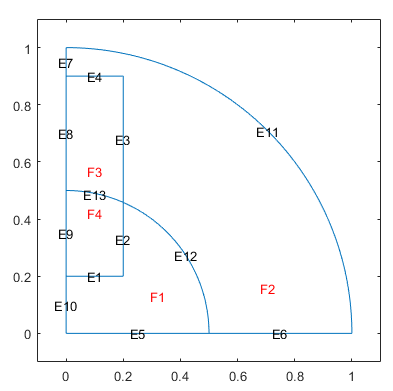# csgdel

Delete boundaries between subdomains

## Syntax

``[dl1,bt1] = csgdel(dl,bt,bl)``
``[dl1,bt1] = csgdel(dl,bt)``

## Description

example

````[dl1,bt1] = csgdel(dl,bt,bl)` deletes the boundaries `bl` between subdomains. If deleting the boundaries in `bl` makes the decomposed geometry matrix inconsistent, then `csgdel` deletes additional border segments (edge segments between subdomains) to preserve consistency.Deleting boundaries typically changes the edge IDs of the remaining boundaries.`csgdel` does not delete boundary segments (outer boundaries).```

example

````[dl1,bt1] = csgdel(dl,bt)` deletes all boundaries between subdomains.```

## Examples

collapse all

Delete edges in a 2-D geometry created in the PDE Modeler app and exported to the MATLAB® workspace.

Create a geometry in the PDE Modeler app by entering the following commands in the MATLAB Command Window:

```pdecirc(0,0,1,"C1") pdecirc(0,0,0.5,"C2") pderect([-0.2 0.2 0.2 0.9],"R1") pderect([0 1 0 1],"SQ1")```

Reduce the geometry to the first quadrant by intersecting it with a square. To do this, enter `(C1+C2+R1)*SQ1` in the Set formula field.

From the PDE Modeler app, export the geometry description matrix, set formula, and name-space matrix to the MATLAB workspace by selecting Export Geometry Description, Set Formula, Labels from the Draw menu.

In the MATLAB Command Window, use the `decsg` function to decompose the exported geometry into minimal regions. This creates an `AnalyticGeometry` object `dl`. Plot `dl`.

```[dl,bt] = decsg(gd,sf,ns); pdegplot(dl,"EdgeLabels","on","FaceLabels","on") xlim([-0.1 1.1]) ylim([-0.1 1.1])```Remove edges 1, 2, and 13 using the `csgdel` function. Specify the edges to delete as a vector of edge IDs. Plot the resulting geometry.

```[dl1,bt1] = csgdel(dl,bt,[1 2 13]); pdegplot(dl1,"EdgeLabels","on","FaceLabels","on") xlim([-0.1 1.1]) ylim([-0.1 1.1])```Now remove all boundaries between subdomains and plot the resulting geometry.

```[dl1,bt1] = csgdel(dl,bt); pdegplot(dl1,"EdgeLabels","on","FaceLabels","on") xlim([-0.1 1.1]) ylim([-0.1 1.1])```## Input Arguments

collapse all

Decomposed geometry matrix, returned as a matrix of double-precision numbers. It contains a representation of the decomposed geometry in terms of disjointed minimal regions constructed by the `decsg` algorithm. Each edge segment of the minimal regions corresponds to a column in `dl`. Edge segments between minimal regions (subdomains) are border segments. Outer boundaries are boundary segments. In each column, the second and third rows contain the starting and ending x-coordinates. The fourth and fifth rows contain the corresponding y-coordinates. The sixth and seventh rows contain left and right minimal region labels with respect to the direction induced by the start and end points (counterclockwise direction on circle and ellipse segments). There are three types of possible edge segments in a minimal region:

• For circle edge segments, the first row is `1`. The eighth and ninth rows contain the coordinates of the center of the circle. The 10th row contains the radius.

• For line edge segments, the first row is `2`.

• For ellipse edge segments, the first row is `4`. The eighth and ninth rows contain the coordinates of the center of the ellipse. The 10th and 11th rows contain the semiaxes of the ellipse. The 12th row contains the rotational angle of the ellipse.

All columns in a decomposed geometry matrix have the same number of rows. Rows that are not required for a particular shape are filled with zeros.

Row number Circle edge segment Line edge segment Ellipse edge segment
1124
2starting x-coordinatestarting x-coordinatestarting x-coordinate
3ending x-coordinateending x-coordinateending x-coordinate
4starting y-coordinatestarting y-coordinatestarting y-coordinate
5ending y-coordinateending y-coordinateending y-coordinate
6left minimal region labelleft minimal region labelleft minimal region label
7right minimal region labelright minimal region labelright minimal region label
8x-coordinate of the center x-coordinate of the center
9y-coordinate of the center y-coordinate of the center
10radius of the circle x-semiaxis before rotation
11  y-semiaxis before rotation
12

Angle in radians between x-axis and first semiaxis

Data Types: `double`

Boolean table relating the original shapes to the minimal regions, returned as a matrix of 1s and 0s.

Data Types: `double`

Boundaries to delete, specified as a positive integer or a vector of positive integers. Each integer represents a boundary ID.

Data Types: `double`

## Output Arguments

collapse all

Modified decomposed geometry matrix, returned as a matrix of double-precision numbers.

Data Types: `double`

Boolean table relating the remaining original shapes to the minimal regions, returned as a matrix of 1s and 0s.

Data Types: `double`

## Version History

Introduced before R2006a